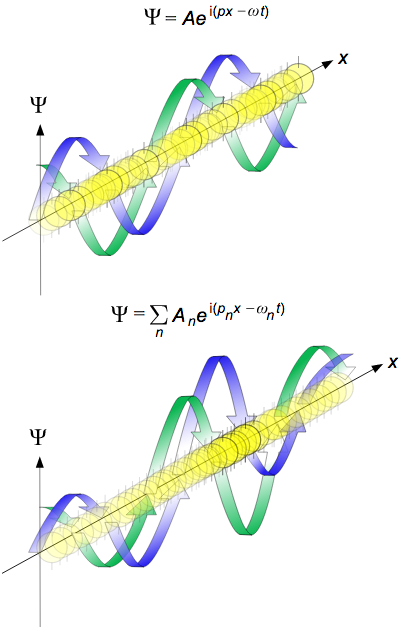## The de Broglie Wavelength

#### Learning Objective

• Use the de Broglie equations to determine the wavelength, momentum, frequency, or kinetic energy of particles

#### Key Points

• At the end of the 19th century, light was thought to consist of waves of electromagnetic fields that propagated according to Maxwell’s equations, while matter was thought to consist of localized particles.
• In his 1905 paper on the photoelectric effect, Albert Einstein postulated that light was emitted and absorbed as localized packets or quanta (now called photons).
• De Broglie showed that the theory of relativity was applicable to not just photons but also all particles with linear momentum, and therefore that matter, including electrons, also has wave-like properties.

#### Terms

• wavelengthThe distance between one peak or trough of a travelling oscillation and the next; it is often designated in physics as λ and corresponds to the velocity divided by the frequency.
• frequencyThe number of occurrences of a repeating event per unit of time.

At the end of the 19th century, light was thought to consist of waves of electromagnetic fields that propagated according to Maxwell’s equations, while matter was thought to consist of localized particles. This division was challenged when, in his 1905 paper on the photoelectric effect, Albert Einstein postulated that light was emitted and absorbed as localized packets or quanta (now called photons). These quanta would have an energy of:

$E=hv$

where v is the frequency of the light and h is Planck’s constant. Einstein’s postulate was confirmed experimentally by Robert Millikan and Arthur Compton over the next two decades. Thus it became apparent that light has both wave-like and particle-like properties. In his 1924 PhD thesis, de Broglie sought to expand this wave-particle duality to all material particles with linear momentum.de Broglie wavesPropagation of de Broglie waves in 1 dimension (the real part of the complex amplitude is blue and the imaginary part is green; top: plane wave, bottom: wave packet.). The probability (shown as the color opacity) of finding the particle at a given point x is spread out like a waveform, with no definite position of the particle. As the amplitude increases above zero the curvature decreases, so the amplitude decreases again, and vice versa – the result is an alternating amplitude: a wave.

In 1926, Erwin Schrödinger published an equation describing how a matter wave should evolve—the matter wave equivalent of Maxwell’s equations—and used it to derive the energy spectrum of hydrogen. That same year, Max Born published his now-standard interpretation that the square of the amplitude of a matter wave gives the probability of finding a particle at a given place. This interpretation was in contrast to de Broglie’s own interpretation, in which the wave corresponds to the physical motion of a localized particle.

## The De Broglie Equations

The de Broglie equations relate the wavelength (λ) to the momentum (p), and the frequency (f) to the kinetic energy (E) (excluding its rest energy and any potential energy) of a particle:

$\lambda ={ h }/p$ and $f={ E }/{ h }$

where h is Planck’s Constant. The two equations can be equivalently written as

$p =\hbar k$ and $E=\hbar \omega$

where $\hbar ={ h }/{ 2\pi }$ is the reduced Planck’s constant,

where $k={ 2\pi }/{ \lambda }$ is the angular wavenumber, and

where $\omega =2\pi f$ is the angular frequency.

De Broglie’s key realization was that in a one-electron atom, for a wave to have a stable amplitude and not decay over time, an integer number (n) of wavelengths must fit into a single circumference drawn by the Bohr orbit. He related this to the principal quantum number n through the equation:

$n\lambda = 2\pi{r}$

This was fortunately reminiscent of Bohr’s observation about the angular momentum of an electron, which had already been established:

$m_{e}vr=2\pi{r}$

By inspection of the above and with minor rearrangement, the de Broglie relation can be obtained as follows:

$\lambda = \frac{h}{m_{e}{v}} = \frac{h}{p}$

## Confirming the de Broglie Hypothesis

In 1927 at Bell Labs, Clinton Davisson and Lester H. Germer fired slow-moving electrons at a crystalline nickel target. The angular dependence of the reflected electron intensity was measured and was determined to have the same diffraction pattern as those predicted by Bragg for X-rays. Before the acceptance of the de Broglie hypothesis, diffraction was a property that was thought to only be exhibited by waves. Therefore, the presence of any diffraction effects by matter demonstrated the wave-like nature of matter. When the de Broglie wavelength was inserted into the Bragg condition, the observed diffraction pattern was predicted, thereby experimentally confirming the de Broglie hypothesis for electrons.

Experiments with Fresnel diffraction and specular reflection of neutral atoms confirm the application of the de Broglie hypothesis to atoms, i.e. the existence of atomic waves that undergo diffraction, interference, and allow quantum reflection by the tails of the attractive potential. Advances in laser cooling have allowed the cooling down of neutral atoms to temperatures near absolute zero. At these temperatures, the thermal de Broglie wavelengths come into the micrometer range. Using Bragg diffraction of atoms and a Ramsey interferometry technique, the de Broglie wavelength of cold sodium atoms was explicitly measured and found to be consistent with the temperature measured by a different method.

Recent experiments even confirm the de Broglie relations for molecules and macromolecules, which are normally considered too large to undergo quantum mechanical effects. In 1999, a research team in Vienna demonstrated diffraction for molecules as large as fullerenes. The researchers calculated a de Broglie wavelength of the most probable C60velocity as 2.5 pm. More recent experiments prove the quantum nature of molecules with a mass up to 6910 amu. In general, the de Broglie hypothesis is expected to apply to any well-isolated object. Even macroscopic objects like tennis balls have a calculable de Broglie wavelength; however, they would be much too small to observe experimentally, and their wave-like nature is not intuitive to common experience.